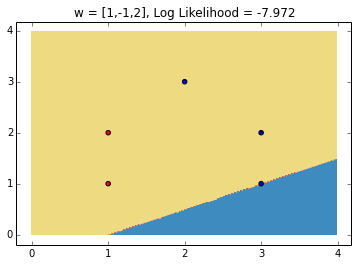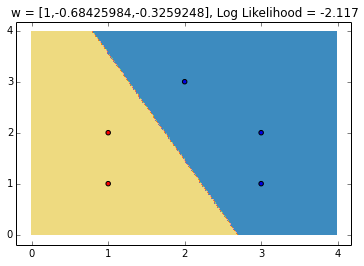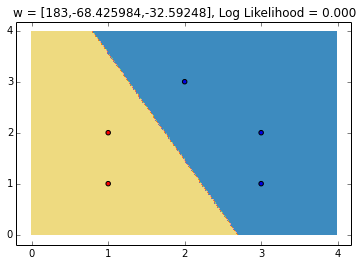# Is Logistic Regression a linear classifier?

A linear classifier is one where a hyperplane is formed by taking a linear combination of the features, such that one 'side' of the hyperplane predicts one class and the other 'side' predicts the other. $$\require{cancel} \require{color} \definecolor{red}{RGB}{255,0,0}$$ For logistic regression, we have that

\begin{align} P(Y=0|X) &= \frac{1}{1 + exp(w_0 + \sum_i{w_iX_i})}\\ P(Y=1 | X) &= 1 - P(Y=0|X) = \frac{exp(w_0 + \sum_i{w_iX_i})}{1 + exp(w_0 + \sum_i{w_iX_i})} \end{align}

We would predict positive if $$P(Y=1 \vert X) > P(Y=0 \vert X)$$, or equivalently:

$\frac{P(Y = 1 | X)}{P(Y= 0|X)} > 1$

Taking logs on both sides (note that the log function is monotonically increasing, meaning that it preserves the order of inputs, and thus inequalities are still valid), we have:

$log\left(\frac{P(Y = 1 | X)}{P(Y= 0|X)}\right) > 0$ $log(exp(w_0 + \sum_i{w_iX_i}) - {\color{red} \cancel{log(1 + exp(w_0 + \sum_i{w_iX_i})}} - \cancel{log(1)} + {\color{red} \bcancel{log(1 + exp(w_0 + \sum_i{w_iX_i})}} > 0$ $w_0 + \sum_i{w_iX_i} > 0 \tag{predict 1 if this happens}$

Thus, we see that the decision boundary is given by the plane $$w_0 + \sum_i{w_iX_i}$$.

### Some example decision boundaries

Note that on the second figure, the log likelihood is higher. This is expected, as the decision boundary separates the data points better than the first one.### The need for regularization

Note in the figure above that the log likelihood is not exacly 0, which would be the case if the likelihood was exactly one, meaning we fit the data perfectly. This is because the log likelihood depends not only on the decision boundary, but on the 'confidence' of each prediction. Also note that we can increase the confidence while maintaining the same decision boundary, by simply scaling up the parameters of w. Note that if the data is linearly separable, the weights would go to infinity. In general, not controling the norm of w will lead to overfitting, which is why we need regularization.Written on March 31, 2015